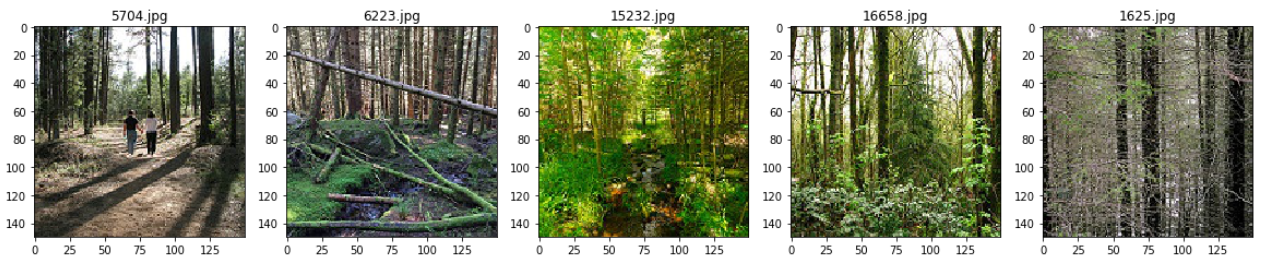Original article was published by Renu Khandelwal on Deep Learning on Medium## A simple guide to different techniques for loading a custom image dataset into deep learning models.

In this article, you will learn how to load and create image train and test dataset from custom data as an input for Deep learning models. You will learn to load the dataset using.

The dataset used here is Intel Image Classification from Kaggle.

Intel Image classification dataset is already split into train, test, and Val, and we will only use the training dataset to learn how to load the dataset using different libraries.

1. Open the image file. The format of the file can be JPEG, PNG, BMP, etc.
2. Resize the image to match the input size for the Input layer of the Deep Learning model.
3. Convert the image pixels to float datatype.
4. Normalize the image to have pixel values scaled down between 0 and 1 from 0 to 255.
5. Image data for Deep Learning models should be either a numpy array or a tensor object.

## The folder structure of the custom image data

Importing required libraries

`import pandas as pdimport numpy as npimport osimport tensorflow as tfimport cv2from tensorflow import kerasfrom tensorflow.keras import layers, Dense, Input, InputLayer, Flattenfrom tensorflow.keras.models import Sequential, Modelfrom  matplotlib import pyplot as pltimport matplotlib.image as mpimg%matplotlib inline`

Printing random five images from one of the folders

`plt.figure(figsize=(20,20))test_folder=r'CV\Intel_Images\seg_train\seg_train\forest'for i in range(5):    file = random.choice(os.listdir(img_folder))    image_path= os.path.join(img_folder, file)    img=mpimg.imread(image_path)    ax=plt.subplot(1,5,i+1)    ax.title.set_text(file)    plt.imshow(img)`

`IMG_WIDTH=200IMG_HEIGHT=200img_folder=r'CV\Intel_Images\seg_train\seg_train\'`

Creating the image data and the labels from the images in the folder

In the function below

• The source folder is the input parameter containing the images for different classes.
• Read the image file from the folder and convert it to the right color format.
• Resize the image based on the input dimension required for the model
• Convert the image to a Numpy array with float32 as the datatype
• Normalize the image array to have values scaled down between 0 and 1 from 0 to 255 for a similar data distribution, which helps with faster convergence.
`def create_dataset(img_folder):    img_data_array=[]    class_name=[]    for dir1 in os.listdir(img_folder):        for file in os.listdir(os.path.join(img_folder, dir1)):            image_path= os.path.join(img_folder, dir1,  file)            image= cv2.imread( image_path, cv2.COLOR_BGR2RGB)            image=cv2.resize(image, (IMG_HEIGHT, IMG_WIDTH),interpolation = cv2.INTER_AREA)            image=np.array(image)            image = image.astype('float32')            image /= 255             img_data_array.append(image)            class_name.append(dir1)    return img_data_array, class_name# extract the image array and class nameimg_data, class_name =create_dataset(r'CV\Intel_Images\seg_train\seg_train')`

Converting text labels to numeric codes

Create a dictionary for all unique values for the classes

`target_dict={k: v for v, k in enumerate(np.unique(class_name))}target_dict`

Convert the class_names to their respective numeric value based on the dictionary

`target_val=  [target_dict[class_name[i]] for i in range(len(class_name))]`

Creating a simple deep learning model and compiling it

`model=tf.keras.Sequential(        [            tf.keras.layers.InputLayer(input_shape=(IMG_HEIGHT,IMG_WIDTH, 3)),            tf.keras.layers.Conv2D(filters=32, kernel_size=3, strides=(2, 2), activation='relu'),            tf.keras.layers.Conv2D(filters=64, kernel_size=3, strides=(2, 2), activation='relu'),            tf.keras.layers.Flatten(),            tf.keras.layers.Dense(6)        ])encoder.compile(optimizer='rmsprop', loss='sparse_categorical_crossentropy', metrics=['accuracy'])`

We finally fit our dataset to train the model. We can use Numpy array as the input

`history = model.fit(x=np.array(img_data, np.float32), y=np.array(list(map(int,target_val)), np.float32), epochs=5)`

We can also convert the input data to tensors to train the model by using tf.cast()

`history = model.fit(x=tf.cast(np.array(img_data), tf.float64), y=tf.cast(list(map(int,target_val)),tf.int32), epochs=5)`

We will use the same model for further training by loading image dataset using different libraries

`from PIL import Image`

Creating the image data and the labels from the images in the folder using PIL

In the function below

• The source folder is the input parameter containing the images for different classes.
• Open the image file from the folder using PIL.
• Resize the image based on the input dimension required for the model
• Convert the image to a Numpy array with float32 as the datatype
• Normalize the image array for faster convergence.
`def create_dataset_PIL(img_folder):    img_data_array=[]    class_name=[]    for dir1 in os.listdir(img_folder):        for file in os.listdir(os.path.join(img_folder, dir1)):            image_path= os.path.join(img_folder, dir1,  file)            image= np.array(Image.open(image_path))            image= np.resize(image,(IMG_HEIGHT,IMG_WIDTH,3))            image = image.astype('float32')            image /= 255              img_data_array.append(image)            class_name.append(dir1)    return img_data_array , class_namePIL_img_data, class_name=create_dataset_PIL(img_folder)`

Converting text labels to numeric codes

Following is the same code that we used for CV2

`target_dict={k: v for v, k in enumerate(np.unique(class_name))}target_val=  [target_dict[class_name[i]] for i in range(len(class_name))]`

Creating and compiling a simple Deep Learning Model

`model=tf.keras.Sequential(        [            tf.keras.layers.InputLayer(input_shape=(IMG_HEIGHT,IMG_WIDTH, 3)),            tf.keras.layers.Conv2D(filters=32, kernel_size=3, strides=(2, 2), activation='relu'),            tf.keras.layers.Conv2D(filters=64, kernel_size=3, strides=(2, 2), activation='relu'),            tf.keras.layers.Flatten(),            tf.keras.layers.Dense(6)        ])encoder.compile(optimizer='rmsprop', loss='sparse_categorical_crossentropy', metrics=['accuracy'])`

We finally fit our dataset to train the model. We can use Numpy array as the input

`history = model.fit(x=np.array(PIL_img_data, np.float32), y=np.array(list(map(int,target_val)), np.float32), epochs=5)`

We can also convert the input data to tensors to train the model by using tf.cast()

`history = model.fit(x=tf.cast(np.array(PIL_img_data), tf.float64), y=tf.cast(list(map(int,target_val)),tf.int32), epochs=5)`

The process is the same for loading the dataset using CV2 and PIL except for a couple of steps.